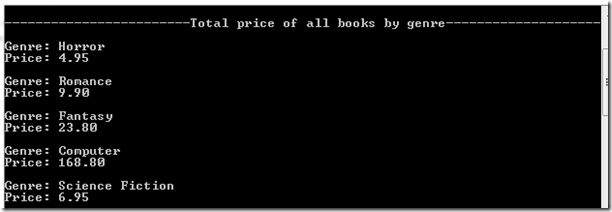Geeks With Blogs
Max's Blog .NET, Windows Phone, SQL Server and other exciting stuff

Now that we’ve got some basics of LINQ for XML. I think we should also look into group by queries in LINQ for XML. My motivation here would be to find the total price of books for each of the genre. For this, I should write LINQ something similar to this below:

`            var query3 = from p2 in query_1`
`                         group p2 by p2.Element("genre").Value into b1`
`                         select new { Value = b1.Key, Price = b1 };`

We group by particular node value using group p2 by p2.Element("genre").Value into b1, here b1.Key will give you the distinct values in that node value by which the values are grouped. b1 as a whole will have a collection of items for each of the key. We can easily iterate through this to find the sum using:

`            foreach (var group in query3)`
`            {`
`                Console.WriteLine("Genre: " + group.Value);`
`                decimal price = 0;`
`                foreach (var grp_Price in group.Price)`
`                {`
`                    price += Convert.ToDecimal( grp_Price.Element("price").Value );`
`                }`
`                Console.WriteLine("Price: " + price.ToString()+"\n");`
`            }`

That is it, now it should give all the genre and total price of books under each genre. The output should be something likeThe final code file for this exercise is here. Please let me have your feedback via the comments below.

Technorati Tags: